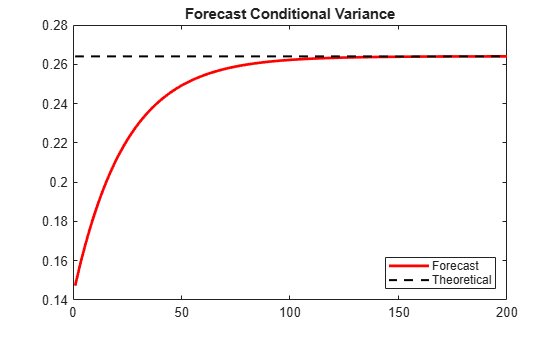Documentation

### This is machine translation

Mouseover text to see original. Click the button below to return to the English version of the page.

## Forecast a Conditional Variance Model

This example shows how to forecast a conditional variance model using `forecast`.

### Load the data and specify the model.

Load the Deutschmark/British pound foreign exchange rate data included with the toolbox, and convert to returns. For numerical stability, convert returns to percentage returns.

```load Data_MarkPound r = price2ret(Data); pR = 100*r; T = length(r);```

Specify and fit a GARCH(1,1) model.

```Mdl = garch(1,1); EstMdl = estimate(Mdl,pR);```
``` GARCH(1,1) Conditional Variance Model (Gaussian Distribution): Value StandardError TStatistic PValue ________ _____________ __________ __________ Constant 0.010868 0.0012972 8.3779 5.3897e-17 GARCH{1} 0.80452 0.016038 50.162 0 ARCH{1} 0.15433 0.013852 11.141 7.9449e-29 ```

### Generate MMSE forecasts.

Use the fitted model to generate MMSE forecasts over a 200-period horizon. Use the observed return series as presample data. By default, `forecast` infers the corresponding presample conditional variances. Compare the asymptote of the variance forecast to the theoretical unconditional variance of the GARCH(1,1) model.

```v = forecast(EstMdl,200,pR); sig2 = EstMdl.Constant/(1-EstMdl.GARCH{1}-EstMdl.ARCH{1}); figure plot(v,'r','LineWidth',2) hold on plot(ones(200,1)*sig2,'k--','LineWidth',1.5) xlim([0,200]) title('Forecast Conditional Variance') legend('Forecast','Theoretical','Location','SouthEast') hold off```The MMSE forecasts converge to the theoretical unconditional variance after about 160 steps.